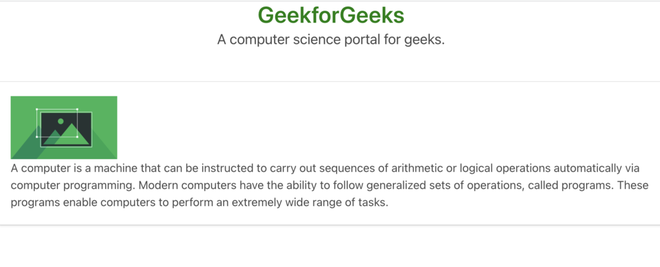# Bulma Image Variables

• Last Updated : 26 Jul, 2022

Bulma is a free and open-source CSS framework based on flexbox. It is component rich, compatible, and well documented. It is highly responsive in nature. It uses classes to implement its design.
The image class is kind of a container since images took a few minutes to load, an image container reserved space for that image so that website’s layout not going to break while image loading or even in image errors.

Syntax:

```<figure class="image">
<img src= "path">
</figure>```

Syntax:

`\$property-name: property-value;`

Variable Used:

• \$dimensions: This variable is used to define the dimension of the image. It holds a string type value and the default value is 16 24 32 48 64 96 128.

Example 1: In the below code, we will make use of the above variable to demonstrate the use of the image.

## HTML

 ` ` `<``html``> ` ` `  `<``head``> ` `    ``<``meta` `charset``=``"utf-8"``> ` `    ``<``meta` `name``=``"viewport"` `          ``content``=``"width=device-width,initial-scale=1"``> ` `    ``<``link` `rel``=``"stylesheet"` `href``= ` `"https://cdn.jsdelivr.net/npm/bulma@0.8.0/css/bulma.min.css"``> ` `    ``<``link` `rel``=``"stylesheet"` `href``=``"style.css"``> ` `    ``<``title``>Bulma Variable ` ` ` ` `  `<``body``> ` `    ``<``center``> ` `        ``<``h1` `class``=``"title"``> ` `            ``GeeksforGeeks ` `        `` ` `        ``<``h3` `class``=``"subtitle"``>A computer science portal for geeks ` `        ``<``figure` `class``=``"image is-5by3"``> ` `            ``<``img` `src``= ` `"https://media.geeksforgeeks.org/wp-content/uploads/20210915115837/gfg3-300x300.png"`  `                 ``alt``=``"GFG"``> ` `        `` ` `    `` ` ` ` ` `

SCSS Code:

```\$dimensions: 250px;
.image{
width:\$dimensions;
}```

Compiled CSS Code:

```.image {
width:250px;
}```

Output:Example 2:  In the below code, we will make use of the above variable to demonstrate the use of the image.

## HTML

 ` ` `<``html``> ` ` `  `<``head``> ` `    ``<``title``>Bulma Image Variable ` `    ``<``link` `rel``=``'stylesheet'` `href``= ` `    ``'https://cdnjs.cloudflare.com/ajax/libs/bulma/0.7.5/css/bulma.css'``> ` ` `  `    ``` `    ``<``script` `src``= ` `    ``'https://cdnjs.cloudflare.com/ajax/libs/font-awesome/5.12.0-2/js/all.min.js'``> ` `    `` ` `    ``<``link` `rel``=``"stylesheet"` `href``=``"style.css"``> ` `    ``<``style``> ` `        ``.title{ ` `            ``color:green; ` `        ``} ` `    `` ` ` ` ` `  `<``body``> ` `    ``<``h1` `class``=``"title has-text-centered"``> ` `        ``GeekforGeeks ` `    `` ` `    ``<``h3` `class``=``"subtitle has-text-centered"``> ` `            ``A computer science portal for geeks. ` `    ``<``br``> ` ` `  `    ``<``div` `class``=``"box"``> ` `        ``<``div``> ` `            ``<``figure` `class``=``"image"``> ` `                 ``<``img` `src``= ` `"https://media.geeksforgeeks.org/wp-content/uploads/20200617121258/gfg-image2.png"``> ` `            `` ` `        `` ` `        ``<``div``> ` `            ``<``p``> ` `                ``A computer is a machine that can be ` `                ``instructed to carry out sequences ` `                ``of arithmetic or logical operations ` `                ``automatically via computer ` `                ``programming. Modern computers have ` `                ``the ability to follow generalized ` `                ``sets of operations, called programs. ` `                ``These programs enable computers to ` `                ``perform an extremely wide range of ` `                ``tasks. ` `            `` ` `        `` ` `    ``     ` ` ` ` `

SCSS Code:

```\$dimensions: 150px;
.image{
max-width:\$dimensions;
}```

Compiled CSS Code:

```.image {
max-width: 150px;
}```

Output:My Personal Notes arrow_drop_up
Recommended Articles
Page :